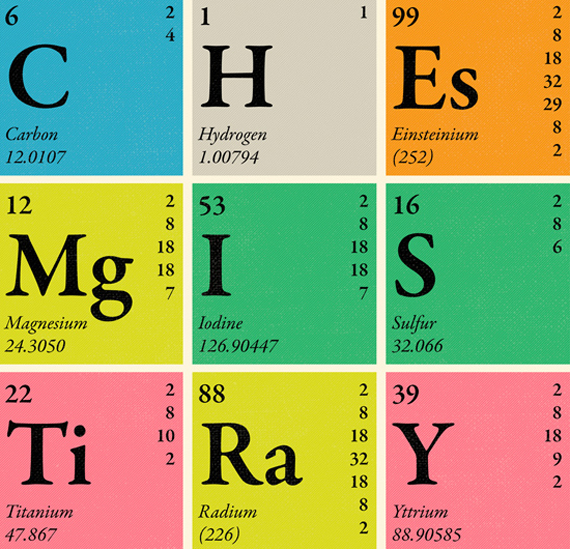Mid Year 8th Grade Science Exam

100 Questions | Total Attempts: 42Settings• 1.
How many chemical bonds can each carbon atom form?
• A.

One

• B.

Two

• C.

Three

• D.

Four

• 2.
A metal washer has a mass of 0.27g and a volume of 0.1 cubic centimeters. What is its density?
• A.

0.027 g/cubic centimeter

• B.

0.037 g/cubic centimeter

• C.

2.7 g/cubic centimeter

• D.

2.7 g/square centimeter

• 3.
Electrons involved in bonding between atoms are...
• A.

Valence electrons.

• B.

Inside the nucleus.

• C.

Closest to the nucleus.

• D.

Positively charged.

• 4.
Normal rainfall is slightly acidic, which means its pH must be...
• A.

Less than 2.

• B.

Between 5-7.

• C.

Between 2-4.

• D.

Between 7-9.

• 5.
Explaining or interpreting the things you observe based on reasoning from what you already know is called...
• A.

Observing

• B.

Inferring

• C.

Predicting

• D.

Classifying

• 6.
The first step in designing or redesigning a product is...
• A.

Thinking about ways to solve the problem.

• B.

Deciding what needs you are trying to meet.

• C.

Building a prototype.

• D.

Fully researching the problem.

• 7.
If you heat a liquid and measure the temperature at which it boils, you are measuring a(n)...
• A.

Atomic property

• B.

Physical property

• C.

Chemical property

• D.

Molecular property

• 8.
If a shoe box measures 6 cm high, 7 cm wide, and 20 cm long, what is its volume?
• A.

420 centimeters

• B.

420 cubic centimeters

• C.

840 cubic centimeters

• D.

840 centimeters

• 9.
In a water solution, how do acids differ from bases?
• A.

Acids form hydrogen ions, while bases form hydroxide ions.

• B.

Acids turn litmus blue, while bases turn litmus red.

• C.

Acids form salts, but bases do not.

• D.

Hydrogen ions remain dissolved, but hydroxide ions do not.

• 10.
A line graph shows...
• A.

Changes in the responding variable only

• B.

Changes in the manipulated variable only

• C.

How the responding variable changes in response to the manipulated variable.

• D.

How the manipulated variable changes on its own.

• 11.
A well-tested explanation for a wide range of observations or experimental results is called a scientific...
• A.

Inquiry.

• B.

Hypothesis.

• C.

Theory.

• D.

Law.

• 12.
What holds atoms together in a molecule?
• A.

Density.

• B.

Gravity.

• C.

Physical bonds.

• D.

Chemical bonds.

• 13.
The number placed below an element's symbol in a chemical formula is called a ...
• A.

Subscript

• B.

Coefficient

• C.

Reactant

• D.

Product

• 14.
One example of a chemical change is...
• A.

Filtering.

• B.

Burning wood.

• C.

Boiling water.

• D.

Crushing a can.

• 15.
You can find the pH of a substance by using...
• A.

Plain paper

• B.

A thermometer.

• C.

Litmus indicator.

• D.

A conductivity tester.

• 16.
What is the goal of technology?
• A.

To produce and deliver cereal.

• B.

To understand how the natural world functions.

• C.

To study the natural world.

• D.

To improve the way people live.

• 17.
Fireworks exploding in the sky and giving off light are an example of a(n)..
• A.

Endothermic change.

• B.

Exothermic change.

• C.

Physical change.

• D.

Change in mass.

• 18.
Which parts of the atom move around the nucleus?
• A.

Atomic mass units

• B.

Electrons

• C.

Protons

• D.

Neutrons

• 19.
Which particles in an atom are in the nucleus?
• A.

Protons and neutrons

• B.

Protons and electrons

• C.

Protons and ions

• D.

Electrons and neutrons

• 20.
When scientists put things into categories or group together items that are alike in some way, they are ....
• A.

Inferring

• B.

Predicting

• C.

Classifying

• D.

Making models

• 21.
Table sugar and table salt are examples of
• A.

Atoms.

• B.

Elements.

• C.

Mixtures.

• D.

Pure substances.

• 22.
The volume of an irregular object can be measured by...
• A.

Multiplying the object's length, width and height.

• B.

Dividing the object's density by its mass.

• C.

Submerging the object in water in a graduated cylinder.

• D.

Placing the object on a triple-beam balance.

• 23.
What is a double bond?
• A.

A bond between two atoms.

• B.

One pair of electrons shared between two atoms.

• C.

Two pairs of electrons shared between two atoms.

• D.

Two pairs of electrons shared between four atoms.

• 24.
What happens when chemical bonds break and new bonds form?
• A.

A physical change

• B.

A chemical reaction

• C.

Matter is destroyed

• D.

Surface area increases

• 25.
Which is a likely use for a base?
• A.

As a vitamin in your food

• B.

Etching metals for printing

• C.

Making foods taste sour

• D.

Making soaps and detergentsBack to top